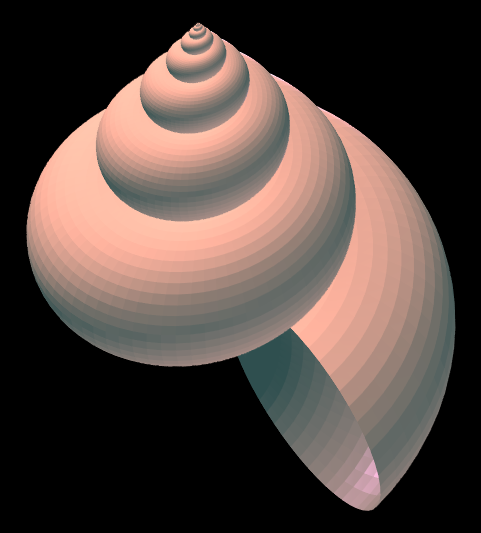# Simulating seashells

In 1838, Rev. Henry Moseley discovered that a large number of mollusk shells and other shells can be described using three parameters: kT, and D.First imagine a thin wire running through the coil of the shell. In cylindrical coordinates, this wire follows the parameterization

r = ekt
z = Tt

If T = 0 this is a logarithmic spiral in the (r, θ) plane. For positive T, the spiral is stretched so that its vertical position is proportional to its radius.

Next we build a shell by putting a tube around this imaginary wire. The radius R of the tube at each point is proportional to the r coordinate: R = Dr.

The image above was created using k = 0.1, T = 2.791, and D = 0.8845 using Øyvind Hammer’s seashell generating software. You can download Hammer’s software for Windows and experiment with your own shell simulations by adjusting the parameters.